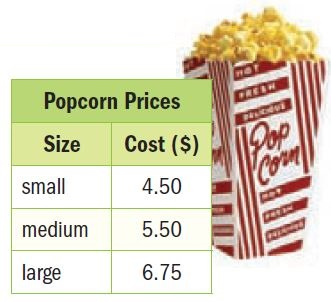Homework Explained - Math Practice 101Dear guest, you are not a registered member. As a guest, you only have read-only access to our books, tests and other practice materials.

As a registered member you can:

Registration is free and doesn't require any type of payment information. Click here to Register.
Go to page:
Chapter 6: Equations and Inequalities; Chapter Review

###• Question 1

A giraffe is 3.5 meters taller than a camel. If a giraffe is 5.5 meters tall, how tall is a camel?

•  $$x=$$
• Question 2

Financial Literacy Martina borrowed $98 from her father. She plans to repay her father at$14 per week. Write and solve an equation to find the number of weeks w required to pay back her father.

•  $$w=$$
• Question 3

A blimp travels 300 miles in 7.5 hours. Assume the blimp travels at a constant speed. Write and solve an equation to find the speed of the blimp. Use the formula d = rt.

•  $$r=$$ $$\text{mph}$$
• Question 4

Joel and two friends went to the movies. They each bought a medium popcorn. Altogether, they spent $45. Write and solve an equation to determine how much each boy spent on a movie ticket?•  $$t=$$ • Question 5 Reason Abstractly Ben is training for football and is lifting 120 pounds on the bench press. He can lift a maximum of 180 pounds. Write and solve an inequality to determine how much additional weight Ben can lift. •  $$\text{up to}$$ $$\text{pounds more}$$ • Question 6 Jessie wants to spend less than$18.75 on new socks. Each pack costs $6. Write and solve an inequality to find the maximum number of packs she can buy. •  $$\text{maximum}$$ $$\text{packs}$$ • Question 7 Financial Literacy Mr. Walker works as a salesperson at an electronics store. He earns$600 a week, plus $50 for every computer he sells. Write and solve an inequality to determine the number of computers Mr. Walker must sell in order to earn at least$1,250 for the week. Interpret the solution.

•  $$c=$$

Yes, email page to my online tutor. (if you didn't add a tutor yet, you can add one here)